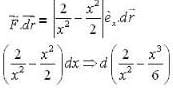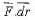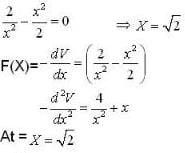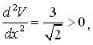For a completely inelastic collision of two p...
For a completely inelastic collision of two particles in one dimension, which of the following statements are correct.
• a)
The kinetic energy is conserved
• b)
The linear momentum is covered
• c)
The particles move with a common velocity after the collision.
• d)
The total energy is conserved.
For a completely inelastic collision of two particles in one dimension...•is exact differential work done by this force is independent of path. Force is conservative.
• Torque about origin is zero . So angular momentum of particle is constant about origin.
• Equilibrium position of particle is given by
F(x)= 0So Potential energy is minimum at X = √ 2
So, x = √2 is point of stable equilibrium
Hence, the particle will move towards x = 1
 1 Crore+ students have signed up on EduRev. Have you?
1 Crore+ students have signed up on EduRev. Have you?

### Learn this topic in detailIIT JAM Physics Mock Test - 4 60 Ques | 180 MinsView courses related to this question

### Quick links for IIT JAM exam850+
Video Lectures2500+
Revision Notes600+
Online Tests34+
Courses
For a completely inelastic collision of two particles in one dimension, which of the following statements are correct.a)The kinetic energy is conservedb)The linear momentum is coveredc)The particles move with a common velocity after the collision.d)The total energy is conserved.Correct answer is option 'B,C,D'. Can you explain this answer?
Question Description
For a completely inelastic collision of two particles in one dimension, which of the following statements are correct.a)The kinetic energy is conservedb)The linear momentum is coveredc)The particles move with a common velocity after the collision.d)The total energy is conserved.Correct answer is option 'B,C,D'. Can you explain this answer? for IIT JAM 2023 is part of IIT JAM preparation. The Question and answers have been prepared according to the IIT JAM exam syllabus. Information about For a completely inelastic collision of two particles in one dimension, which of the following statements are correct.a)The kinetic energy is conservedb)The linear momentum is coveredc)The particles move with a common velocity after the collision.d)The total energy is conserved.Correct answer is option 'B,C,D'. Can you explain this answer? covers all topics & solutions for IIT JAM 2023 Exam. Find important definitions, questions, meanings, examples, exercises and tests below for For a completely inelastic collision of two particles in one dimension, which of the following statements are correct.a)The kinetic energy is conservedb)The linear momentum is coveredc)The particles move with a common velocity after the collision.d)The total energy is conserved.Correct answer is option 'B,C,D'. Can you explain this answer?.
Solutions for For a completely inelastic collision of two particles in one dimension, which of the following statements are correct.a)The kinetic energy is conservedb)The linear momentum is coveredc)The particles move with a common velocity after the collision.d)The total energy is conserved.Correct answer is option 'B,C,D'. Can you explain this answer? in English & in Hindi are available as part of our courses for IIT JAM. Download more important topics, notes, lectures and mock test series for IIT JAM Exam by signing up for free.
Here you can find the meaning of For a completely inelastic collision of two particles in one dimension, which of the following statements are correct.a)The kinetic energy is conservedb)The linear momentum is coveredc)The particles move with a common velocity after the collision.d)The total energy is conserved.Correct answer is option 'B,C,D'. Can you explain this answer? defined & explained in the simplest way possible. Besides giving the explanation of For a completely inelastic collision of two particles in one dimension, which of the following statements are correct.a)The kinetic energy is conservedb)The linear momentum is coveredc)The particles move with a common velocity after the collision.d)The total energy is conserved.Correct answer is option 'B,C,D'. Can you explain this answer?, a detailed solution for For a completely inelastic collision of two particles in one dimension, which of the following statements are correct.a)The kinetic energy is conservedb)The linear momentum is coveredc)The particles move with a common velocity after the collision.d)The total energy is conserved.Correct answer is option 'B,C,D'. Can you explain this answer? has been provided alongside types of For a completely inelastic collision of two particles in one dimension, which of the following statements are correct.a)The kinetic energy is conservedb)The linear momentum is coveredc)The particles move with a common velocity after the collision.d)The total energy is conserved.Correct answer is option 'B,C,D'. Can you explain this answer? theory, EduRev gives you an ample number of questions to practice For a completely inelastic collision of two particles in one dimension, which of the following statements are correct.a)The kinetic energy is conservedb)The linear momentum is coveredc)The particles move with a common velocity after the collision.d)The total energy is conserved.Correct answer is option 'B,C,D'. Can you explain this answer? tests, examples and also practice IIT JAM tests.Explore Courses for IIT JAM exam